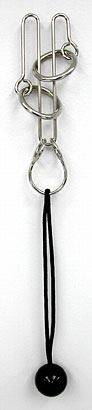# Extending a transformation from a curve to the whole space

• maze

#### maze

Supposing I have a curve, $\vec{f}\left(s,t\right)$ that lives in $\Re^{3}$ and is deforming over time but never intersecting itself (s is the curve parameter and t is time). I would like to extend the deformation of the curve to the entire space around the curve, creating a transformation of the whole space $\vec{g}\left(\vec{x},t\right)$ that is continuous and doesn't overlap itself.

It would be fine to restrict the curve to a series of connected straight line segments, if that would make it easier.

If I can construct such a function, g, I believe I can use it to create a very general method of solving "tanglement puzzles", where the object is to remove a flexible loop of string from a metal contraption.

Here are some pictures that describe what I am taking about.
http://img381.imageshack.us/img381/1968/pathcurvingsmww1.png [Broken]

and here is the function I want to construct:
http://img162.imageshack.us/img162/7487/pathcurvingcoordssmcz2.png [Broken]

And here are a couple examples of "remove the string" tanglement puzzles:http://www.puzzles.ca/puzzle_data_3/xastroknot_l.jpg

Last edited by a moderator:
There are an infinite number of ways of doing that. What additional restrictions do you want to add in order to make that problem unique?

Yes definitely, there are infinitely many legit possibilities for such transformations. Any will do, so long as I can construct it for any curve. I'm interested in a construction, not an existence proof! The simpler or easier to compute, the better.

I was running through some simple ideas such as linear interpolation with tetrahedra, distance weighting, and so forth, but my constructions keep having problems with overlapping for certain curves.

Ahh, I believe I may have a way to do this.

Restrict the curves in question to those that consist of straight line segments, as that is acceptable for my purposes. Next create a cubic lattice of gridpoints in the space. Then, using the curve and a bounding box as boundary conditions, force each grid point position to be the average of the position of all it's neighbors.

I think this should work, though I am not 100% sure and will need to prove it...

Last edited:
Or more generally where the curve isn't straight lines, g is given by the vector laplace equation in each coordinate, using the transform on the curve, f, as a boundary condition. Now I will need to review how to calculate greens functions numerically...